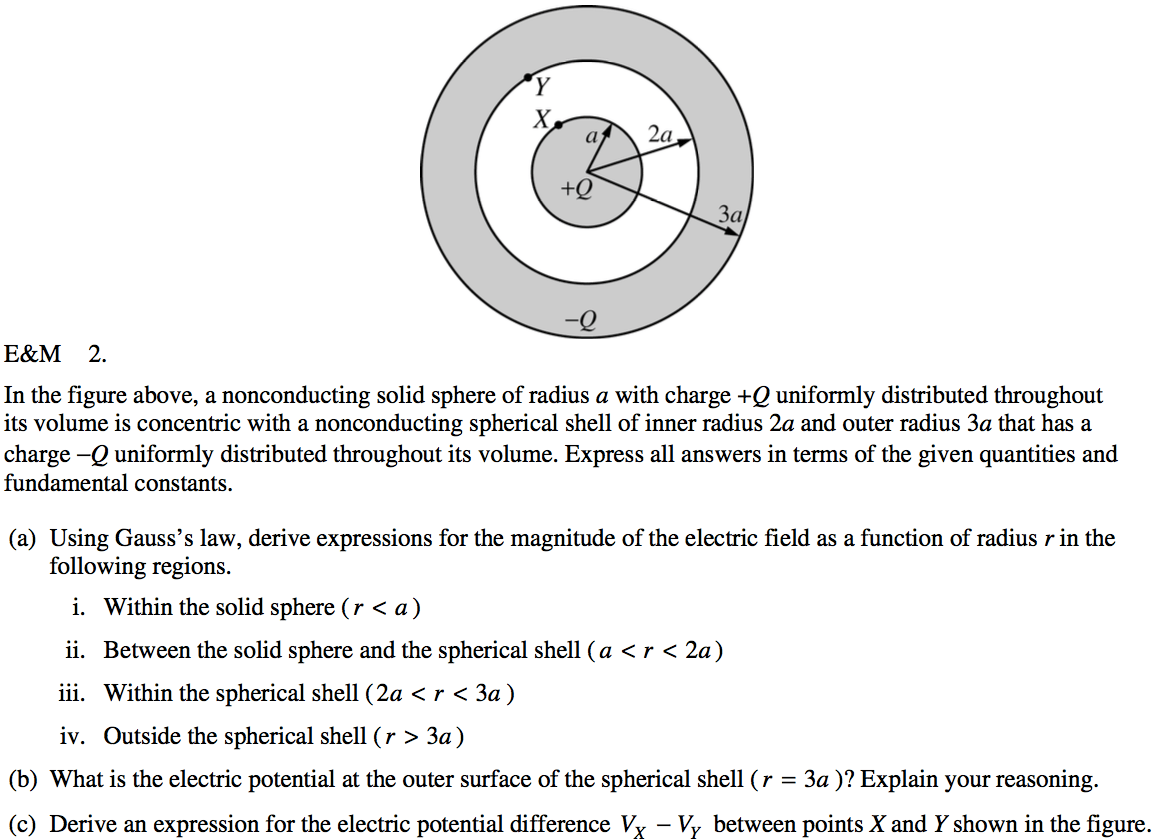# Potential Due to a Charged Ring• Find the electric potential on the axis of a uniformly charged ring of radius R and total charge Q at point P located a distance z from the center of the ring

•••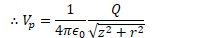# Potential Due to a Charged Disk• Find the electric potential on the axis of a uniformly charged disk of radius R and total charge Q at point P located a distance z from the center of the ring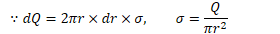# Potential Due to a Spherical Shell of Charge• Find the electric potential both inside and outside a uniformly charged shell of radius R and total charge Q

•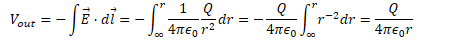•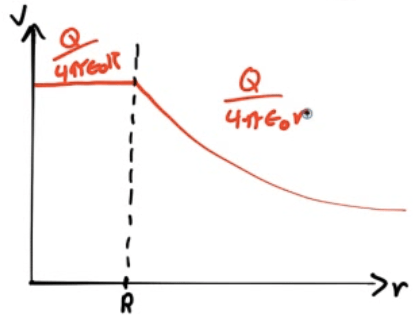# Potential Due to a Uniform Solid Sphere• Find the electric field and electric potential inside a uniformly charged solid insulating sphere of radius R and total charge Q

••••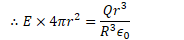••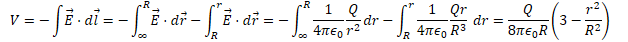# 2012 Free Response Question 1# 2010 Free Response Question 1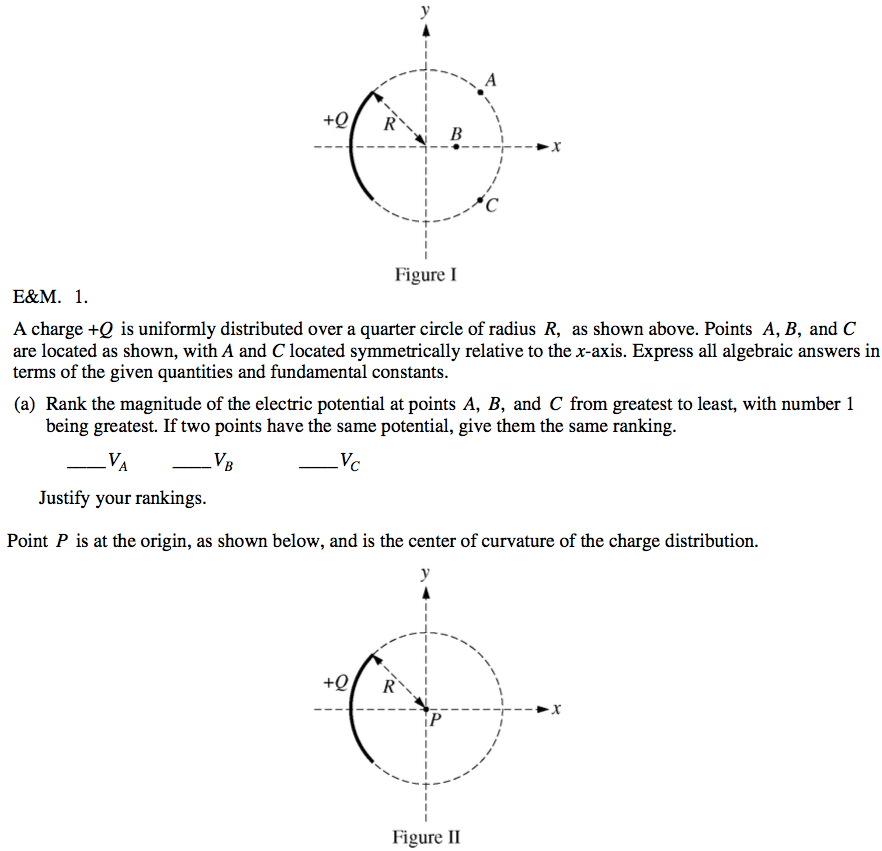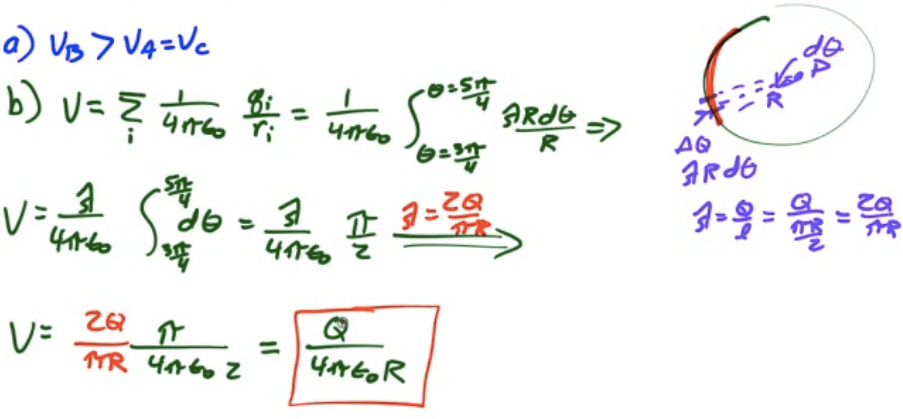# 2009 Free Response Question 1

![A spherically symmetric charge distribution has net positive charge Q) distributed within a radius of R. Its electric potential V as a function of the distance r from the center of the sphere is given by the following. V(r) = 2 -2+3 for r < R 41t€0R V(r) = for r > R 41t€or Express all algebraic answers in terms of the given quantities and fundamental constants. (a) For the following regions, indicate the direction of the electric field E(r) and derive an expression for its magnitude. Radially inward ii. r > R Radially inward Radially outward Radially outward (b) For the following regions, derive an expression for the enclosed charge that generates the electric field in that region, expressed as a function of r. ii. r

R (c) Is there any charge on the surface of the sphere (r = R) ? Yes No If there is, determine the charge. In either case, explain your reasoning. ](./media/image150.png)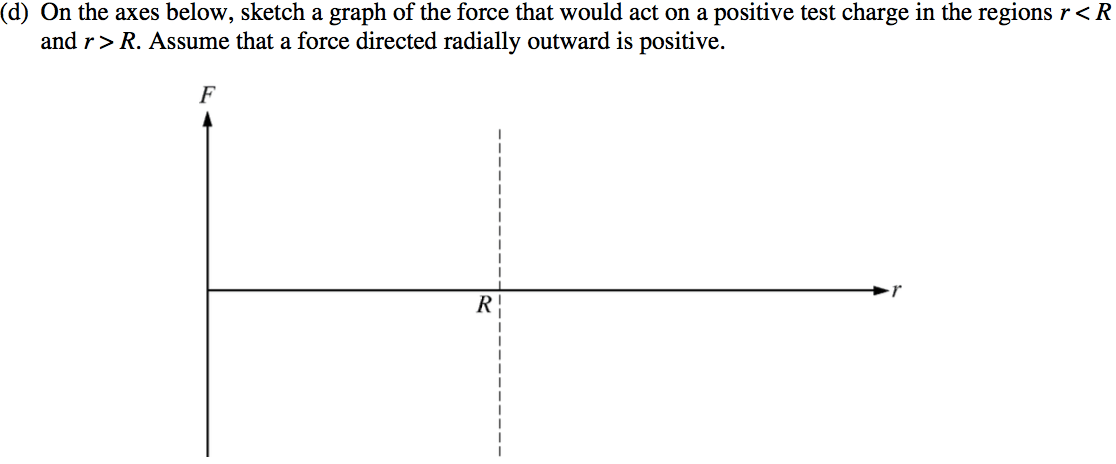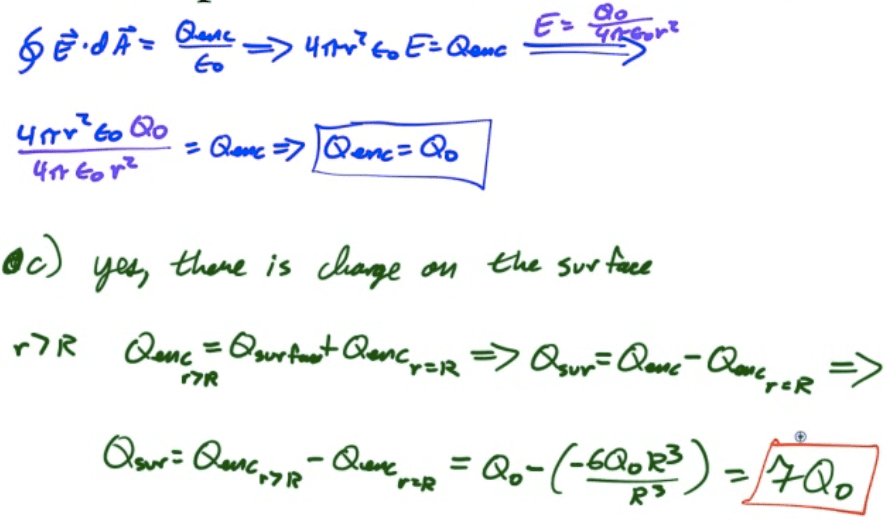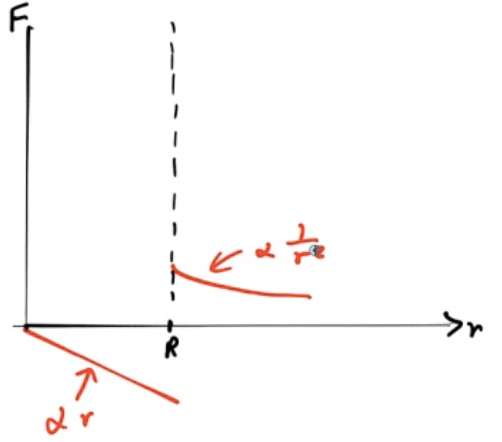# 2007 Free Response Question 2